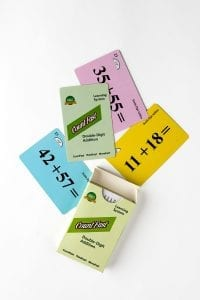####OVERVIEW & PURPOSE

Week four of the 2nd Grade CountFast program focuses two general strategies for adding double-digit numbers with quick mental calculation.  (Addition with no carrying over, addition with carrying forward).  Students will also learn how to quickly solve problems that add up to a multiple of 10.  Card decks should go home with students each day for additional practice with a parent at home. Each week, a new deck is introduced and the previous deck is for the student to keep at home for continued practice.

#### EDUCATION STANDARDS

1. NCTM Standard: develop fluency with basic number combinations for addition and subtraction
2. NCTM Standard: understand the effects of adding and subtracting whole numbers
3. CCSS.MATH.CONTENT.2.NBT.B.5
Fluently add and subtract within 100 using strategies based on place value, properties of operations, and/or the relationship between addition and subtraction.

#### OBJECTIVES

1. Develop strategies for fluency in solving double-digit addition equations using the ‘carry forward’ and ‘no carrying’ concepts.

DAY 1:

Have your child use the YELLOW cards in the deck to explain how to quickly add double-digit numbers when there is no need to ‘carry-over’.  Students turn over one card at a time and solve the problem as fast as they can, being sure to explain to you how he/she arrived at the answer.  For example, 11 + 12 = 23 can be explained in this way: “Two tens and three ones make twenty-three.  I did not need to carry over.” Of course, there are other methods students can use for quick mental calculation.  Some students may find it faster to decompose the numbers and add together those smaller parts. (11 + 12 can be thought of as 10 + 1 + 10 + 2, or 10 + 10 + 1 + 2).  Encourage your child to use the fastest mental calculation method for his/her way of thinking.  There are “Time Record Cards” at the back of this pack if you wish to time your child each time they go through the cards.

DAY 2:

Have your child use the BLUE cards in the deck to explain how to quickly add double-digit numbers in which the one’s numbers add up to exactly 10.  When the one’s digits add up to exactly 10, they can quickly solve the problem by carrying 1 into the ten’s group, adding the ten’s digits, and putting a zero behind that number for the final answer.  For example, 25 + 45 can be solved by quickly noticing that the two 5’s in the one’s places add up to exactly 10.  Add 1 to the ten’s digits (1 + 2 + 4 =7) and then put a zero behind that 7 to make a total of 70.  Practice this thought process several times together.  With repetition of the process, students will get faster and faster mentally calculating what they see with their eyes.  Say the process out loud together with each blue card.

DAY 3: